# Real number calculator

## Result:

### 6 = 6

The calculator makes basic and advanced operations with real numbers, natural numbers, integers, rational and irrational numbers. It also shows detailed step-by-step information about calculation procedure. Solve problems with two, three, or more real numbers in one expression. Add, subtract, and multiply real numbers step-by-step. This calculator performs addition, subtraction, multiplication, or division for calculations on positive or negative real numbers. This online real number calculator will help you understand how to add, subtract, multiply, or divide real numbers.

Real numbers are numbers that can be found on the number line. This includes the natural numbers( 1,2,3 ...), integers (-3) rational (fractions) and irrational numbers (like √2 or π). Positive or negative, large or small, whole numbers or decimal numbers are all real numbers. Imaginary numbers and complex numbers cannot be draw in number line, but in complex plane.

#### Examples:

addition of real numbers: -3/2 + pi/2
subtraction of real numbers: 32.14 - 67.32
parenthesis: sin(pi/2) * (1.1+2.2+3.3)
Complex example: Priority of operators and brackets: 3⋅[8−(−1) + (−3)÷(−3)]^2

## Real numbers in word problems:

• Evaluate 18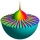Evaluate the expression (-4-7i)-(-6-9i) and write the result in the form a+bi (Real + i* Imaginary).
• The diameter 2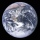The diameter of the Earth is 12,756 kilometers. If Mercury's diameter is about 7,876.6 kilometers shorter than that of the Earth, what is the diameter of Mercury?
• Is equal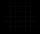Is equal following terms? -9 21 = (-9) 21
• Is complexAre these numbers 2i, 4i, 2i + 1, 8i, 2i + 3, 4 + 7i, 8i, 8i + 4, 5i, 6i, 3i complex?
• Fruit punch 2Alberto makes fruit punch with 24.2 ounces of apple juice and 18.4 ounces of pineapple juice. He splits the fruit punch equally into 6 glasses. How much fruit punch is in each glass?
• Imaginary numbersFind two imaginary numbers whose sum is a real number. How are the two imaginary numbers related? What is its sum?
• Write 3Write a real-world problem involving the multiplication of a fraction and a whole number with a product that is between 8 and 10, then solve the problem.
• The sum 15The sum of the real numbers x and y is 24. Their difference is 12. What is the value of xy?
• Subtract 4Subtract the following decimals. a. 0.98 - 0.053 b. 0.67 - 0.4 c. 0.3 - 0.002 d. 3.2 - .789 e. 6.53 - 4.298 f. 6 - 4.32 g. 7 - 3.574 h. 4.83 - 1.8 i. 3.7 - 1.8 j. 16.17 - 11.632
• Equation with abs value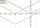How many solutions has the equation ? in the real numbers?
• Logarithmic equation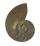Solve equation: log13(7x + 12) = 0
• The sum 10The sum of three decimals are 938.629. Two of them are 456.54 and 392.69. Find the third one.
• Sorting ASCWhich group of decimals is in order from least to greatest? A) 1.04, 0.39, 0.8, 2.1, 0.09 B) 2.1, 1.04, 0.39, 0.8, 0.09 C) 0.09, 0.39, 0.8, 1.04, 2.1 D) 0.09, 0.39, 0.8, 2.1, 1.04
• Sum and productThe sum of the real numbers x and y is 12. Their difference is 8. What is the value of xy?
• Coordinate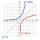Determine missing coordinate of the point M [x, 120] of the graph of the function f bv rule: y = 5xDetermine the numbers b, c that the numbers x1 = -1 and x2 = 3 were roots of quadratic equation: -3x 2 + b x + c = 0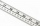Marlon drew a scale drawing of a summer camp. In real life, the sand volleyball court is 8 meters wide. It is 4 centimeters wide in the drawing. What is the drawing's scale factor? Simplify your answer and write it as a ratio, using a colon.